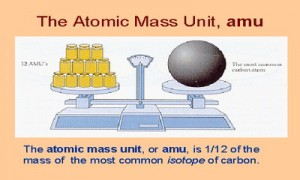Chemistry

# What is Atomic Mass Unit?

Atomic Mass Unit is defined as a mass exactly equal to one-twelve the mass of one carbon-12 atom. One atomic mass is also called one Dalton (Da) or unified atomic mass unit (u).

Mass of one mole of C-12 atoms = 12g = mass of (6.022 * 10^23) C-12 atoms.

1 amu = 1 gram per mole = 1/Na = 1/(6.022 * 10^23) = (1.66 * 10^-24) gram.

In a word: “An atomic mass unit (symbolized AMU or amu) is defined as precisely 1/12 the mass of an atom of carbon-12. The carbon-12 (C-12) atom has six protons and six neutrons in its nucleus”.Examples of Atomic Mass Unit

• A hydrogen-1 atom has a mass of 1.0078250 u (1.0078250 Da).
• By definition, a carbon-12 atom has a mass of 12 u (12 Da).
• A molecule of acetylsalicylic acid (Aspirin) has a mass of 180.16 u (180.16 Da).
• Titin, the largest known protein, has an atomic mass of 3-3.7 megadaltons (3000000 Da).